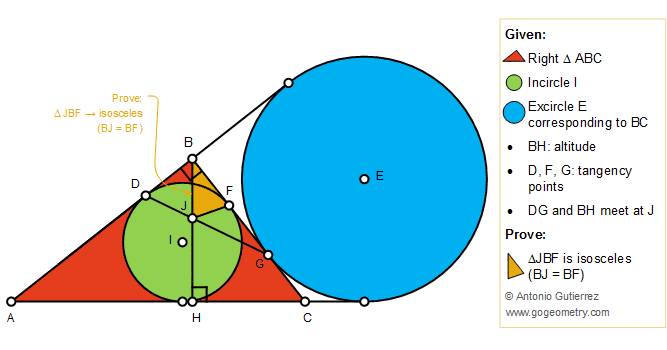# Geometry Problem 1414: Right Triangle, Altitude, Incircle, Excircle, Tangency Points, Isosceles Triangle

### Proposition

The figure below shows a right triangle ABC (angle B = 90 degree) with the altitude BH. The incircle I is tangent to AB, BC, and AC at D, F, and H, respectively. The excircle E corresponding to BC is tangent to BC at G. DG and BH meet at J. Prove that the triangle JBF is an isosceles triangle (BJ = BF).### Geometric Art: Isolines of Problem 1414